# Modelling of Newtons law of cooling in MATLAB

June 3, 2022

By  Shubham

lang: en_US

Sir Isaac Newton, the great English physicist, and mathematician, independently invented differential and integral calculus. He discovered many basic physical laws. The law of cooling is one such physical law. The law states:

The rate of temperature change of a body is directly proportional to the difference in temperatures between the body and its surroundings.

When newton’s law is stated in terms of temperature difference, it results in an ordinary differential equation expressing temperature difference as a function of time. The solution to the ordinary differential equation describes an exponential decrease of temperature difference over time.

## Mathematical representation

Newton’s law of cooling is expressed as,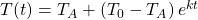Where,is the temperature of body at time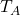is the surrounding temperature of the body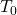is the initial temperature of the body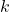is the proportionality constant

Newton did not initially state his law in the above form in 1701. This final simplest version of the law, given by Newton himself, was partly due to confusion in his time between the concepts of heat and temperature, which would not be fully disentangled until much later.

Now that you know what the equation looks like let’s derive it ourselves.

## Derivation of Newton’s law of cooling

Letbe the temperature inside the building andthe outside temperature. Then according to newton’s law,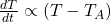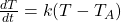Whereis the proportionality constant.

The above ODE will be solved using the variable separable method. On doing separation, integration, and taking exponents, the general solution is,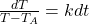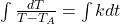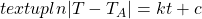Let the initial temperature at time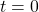be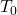and final time temperature at time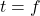be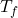, then,Substituting the value of c in general solution,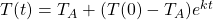## Setting up the model in MATLAB

Code & Report!

Newton's law of cooling describes the relationship between the cooling rate of a system according its environmental temperature. Learn the mathematics behind the law and how it can be modelled; Developed in MATLAB R2021b

To calculate and plot the final temperature, let’s create a function that will do this task for us.

%%%%%%%%%%%%%%%%%%%%%%%%%%%%%%%%%%%%%%%%%%%%%
% Author: Shubham Kumar, MATLAB Helper
%Topic: Newton’s law of cooling
%Website: https://matlabhelper.com
%Date: 18-04-2022
%Version: R2021b
%%%%%%%%%%%%%%%%%%%%%%%%%%%%%%%%%%%%%%%%%%%%%

function [ ] = newton_cooling(k, N, tMax, T_0, T_env)

The input arguments of this function are:

k             -> Cooling constant [1/min]

N            -> Number of time steps

tMax      -> Time interval for simulation

T_0        -> Initial temperature of system

T_env    -> Temperature of surrounding environment

In the initialization part of the function, first set a value for a time from 0 to N having a time step of the time interval that we set up for simulation (tMax) between them. We also need a time increment variable that should have a value of the difference between t(2) and t(1). Use the cooling constant ‘k’ with the time increment variable to set the constant cooling values for all values of time t.

To calculate the final temperature, introduce a for loop that will iterate through every time step. Use the equation that we derived in the earlier section, except here, the loop will go through every value of time step N and calculate the final temperature value for each value of time.

Note: If you are trying to show the result in the console, then assign the last calculated value of the final temperature to another variable and show the result.

The calculated result of our function will be displayed in console. I have included some code that will display the result nicely.

disp('   ');
disp('All temperature in degree Celsius: ');
fprintf('Cooling constant               k  = %2.3e   [1/min]  \n',k);
fprintf('Number of time steps           N  = %4.0f  \n',N);
fprintf('Time interval for simulation   tMax  = %4.0f   [min]  \n',tMax);
fprintf('Environmental temperature      Tenv  = %4.2f   [deg C]  \n',T_env);
fprintf('Initial temperature of system  T0  = %4.2f   [deg C]  \n',T_0);
fprintf('Final temperature of system    Tend  = %4.2f   [deg C]  \n',Tend);

The only thing that remains now is to display the result in a graphical format. You can also set() function in your plot. The x-axis should be time and y-axis should be system temperature that we calculated. The system temperature is the final temperature of the system. You can use certain properties of plot() function to make the graph look nice.

figure(1)
set(gcf,'units','normalized','position',[0.01 0.2 0.22 0.25]);
hold on   xP = t; yP = T;   plot(xP,yP,'b','lineWidth',2);
xP = t; yP = T_env .* ones(1,N);
plot(xP,yP,'g','lineWidth',1);
xlabel('time  t  [min]')
ylabel('system   T   [deg C]');
box on;   grid on;   set(gca,'fontsize',14);

## Analysing the result

Let’s run our function with the following arguments:

newton_cooling(0.05,500,60,100,25)

Here, k is 0.05; the time step is 500, the time interval of simulation is 60 minutes, the initial temperature of the body/system is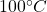, and the surrounding temperature is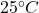.

On running the code with the given input arguments, you should get the following result in the console:

>> newton_cooling(0.05,500,60,100,25)   All temperature in degree Celsius:    Cooling constant               k  = 5.000e-02   [1/min]     Number of time steps           N  =  500     Time interval for simulation   tMax  =   60   [min]     Environmental temperature      Tenv  = 25.00   [deg C]     Initial temperature of system  T0  = 100.00   [deg C]     Final temperature of system    Tend  = 28.70   [deg C]

Along with this result, MATLAB will also generate a graph like the following one,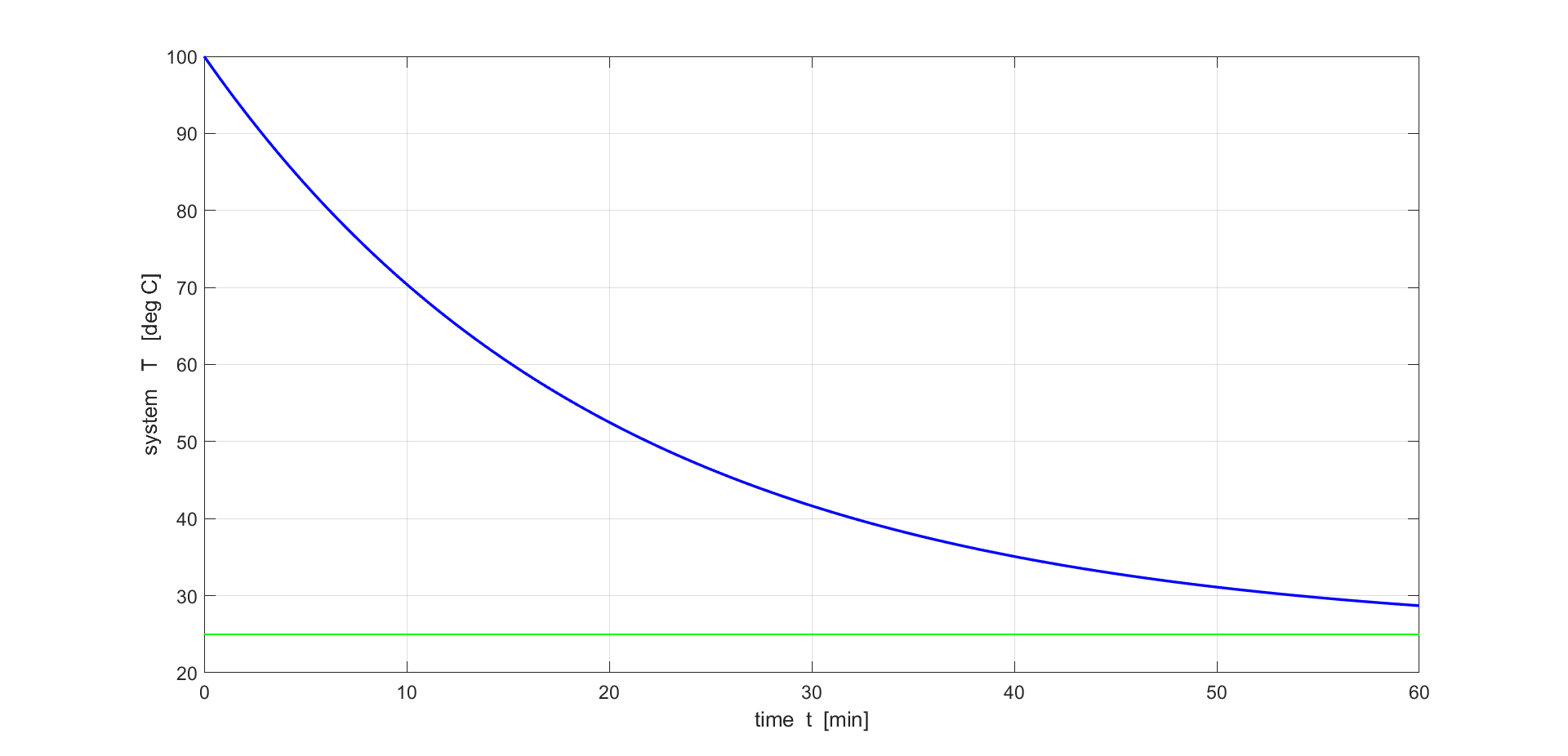Exponential decrease of temperature from 100 degree to 28.70 degree Celsius

Let’s run the program with some more input arguments,

>> newton_cooling(0.05,500,75,156.5,30)  All temperature in degree Celsius:   Cooling constant               k  = 5.000e-02   [1/min]    Number of time steps           N  =  500    Time interval for simulation   tMax  =   75   [min]    Environmental temperature      Tenv  = 30.00   [deg C]   Initial temperature of system  T0  = 156.50   [deg C]    Final temperature of system    Tend  = 32.93   [deg C]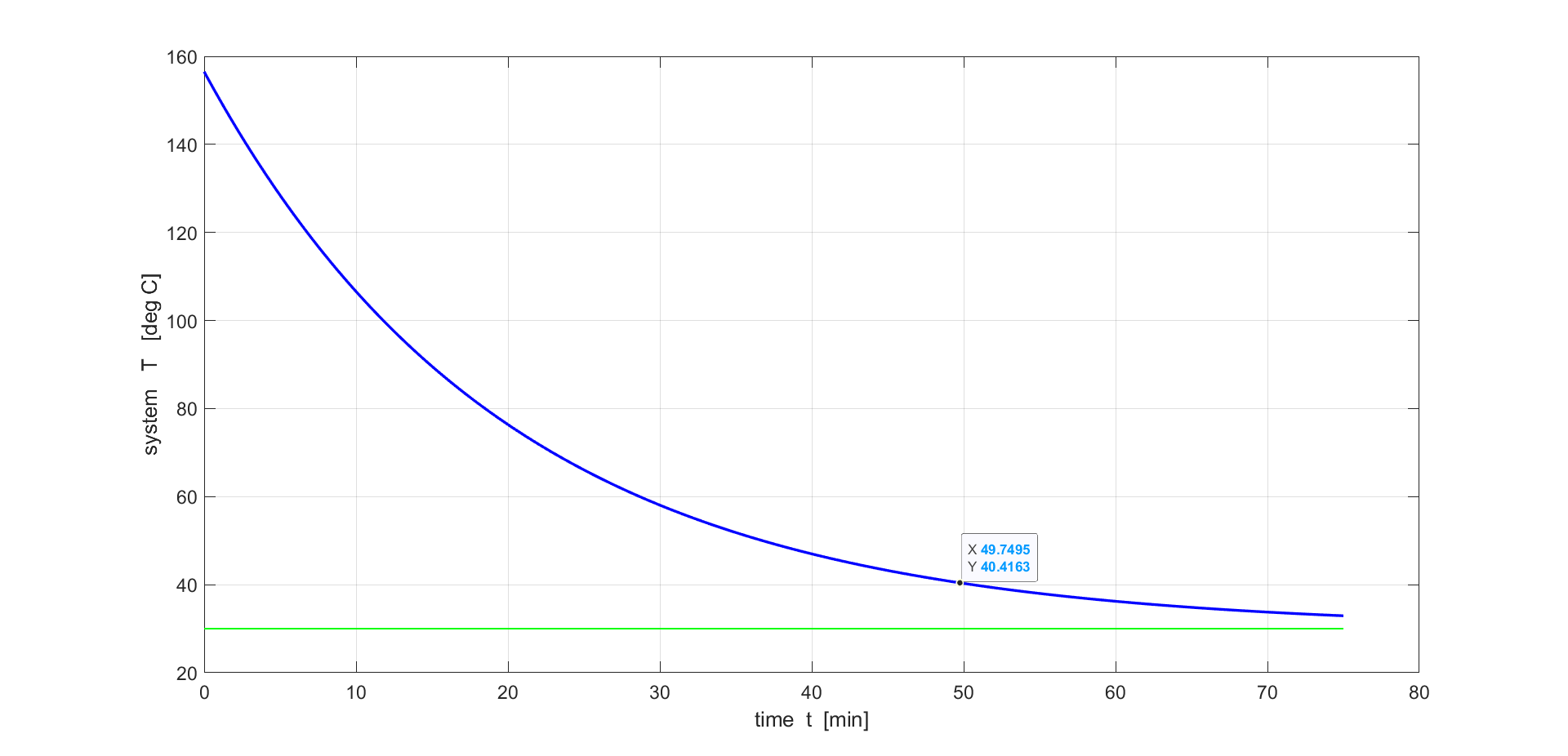Exponential decrease of temperature from 156.5 degree to 32.93 degree Celsius

We can see that the graphs show the exponential decay, or we can say that temperature decreases exponentially over time. The graph verifies that the output temperature is correct as per the formula we derived earlier. Now, let’s check whether our code works when we have negative cooling or when the temperature increases. To do so, run the code with the input arguments where the initial temperature of the system is less than the surrounding temperature. For example,

>> newton_cooling(0.05,500,60,30,150)  All temperature in degree Celsius:   Cooling constant               k  = 5.000e-02   [1/min]    Number of time steps           N  =  500    Time interval for simulation   tMax  =   60   [min]    Environmental temperature      Tenv  = 150.00   [deg C]   Initial temperature of system  T0  = 30.00   [deg C]    Final temperature of system    Tend  = 144.08   [deg C]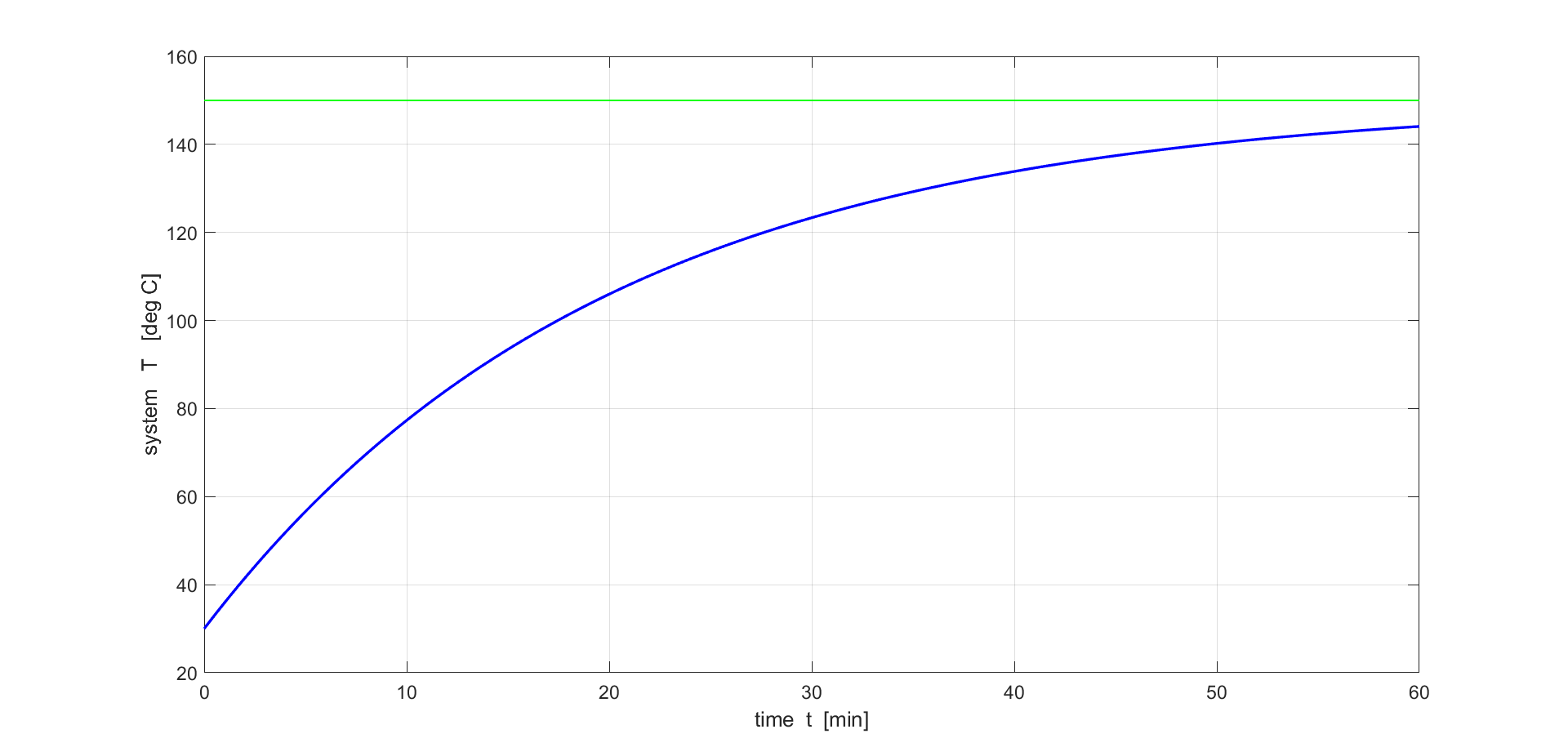Exponential increase of temperature from 30 degree to 144.08 degree Celsius

The graph thus obtained shows an exponential increase in temperature. Hence, our code is valid for decreasing as well as increasing temperature.

## Application of Newton’s law of cooling

The law has uses in predicting the time taken for a hot object to cool down at a constant temperature. Newton’s law is also used in the forensic study of dead bodies as it helps to indicate the death time given by the probable body temperature at the death time and the current body temperature. It also finds uses in studying the first-order transient response of lumped capacitance objects.

Ready to take your MATLAB skills to the next level? Look no further! At MATLAB Helper, we've got you covered. From free community support to expert help and training, we've got all the resources you need to become a pro in no time. If you have any questions or queries, don't hesitate to reach out to us. Simply post a comment below or send us an email at [email protected].

And don't forget to connect with us on LinkedIn, Facebook, and Subscribe to our YouTube Channel! We're always sharing helpful tips and updates, so you can stay up-to-date on everything related to MATLAB. Plus, if you spot any bugs or errors on our website, just let us know and we'll make sure to fix it ASAP.

Ready to get started? Book your expert help with Research Assistance plan today and get personalized assistance tailored to your needs. Or, if you're looking for more comprehensive training, join one of our training modules and get hands-on experience with the latest techniques and technologies. The choice is yours – start learning and growing with MATLAB Helper today!

Education is our future. MATLAB is our feature. Happy MATLABing!

#### Shubham

I have done my bachelor degree in electronics from University of Delhi. I like to tinker with the hardware to understand how it actually works.

#### MATLAB Helper ®

Use Website Chat or WhatsApp at +91-8104622179